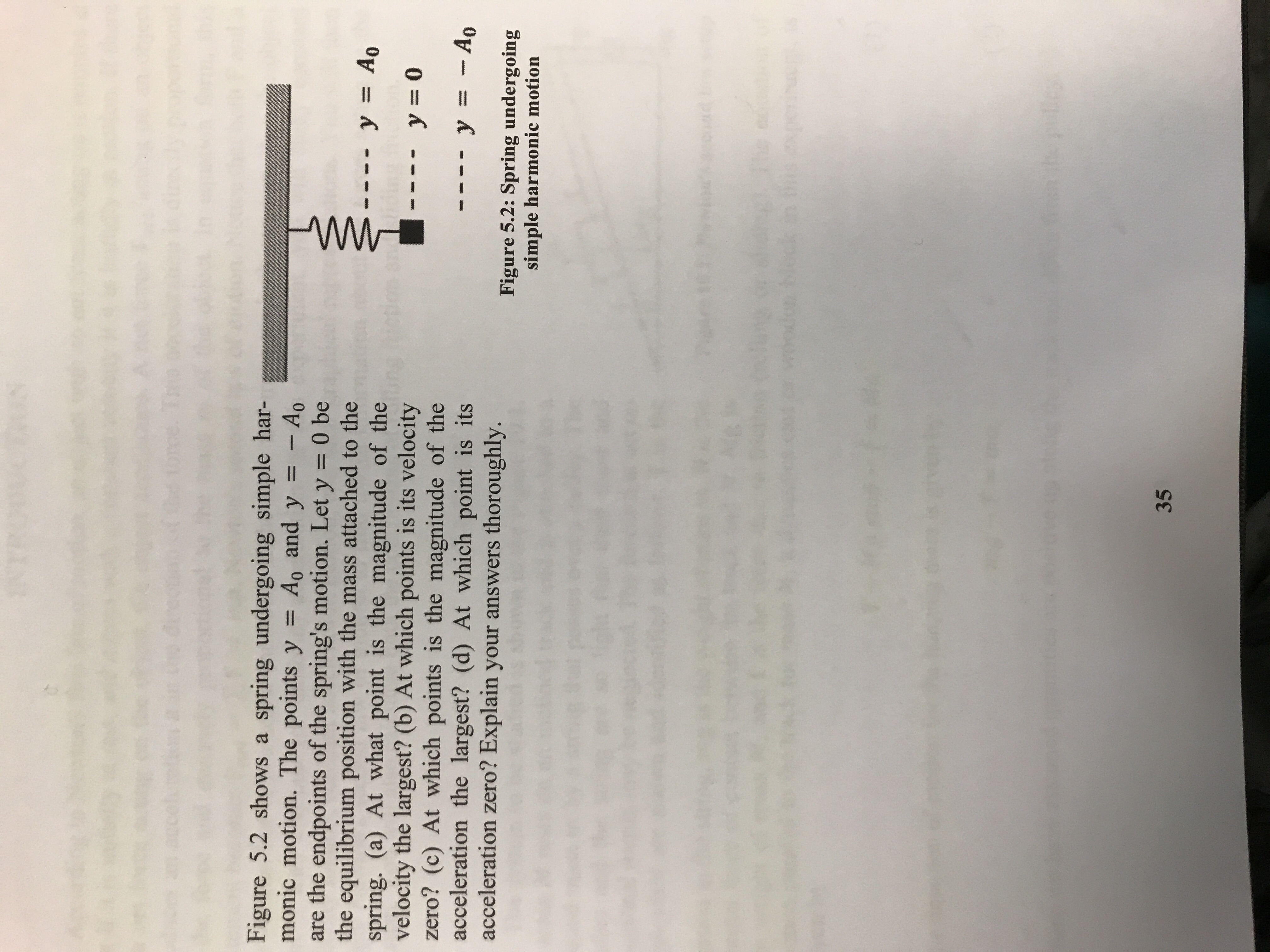# Figure 5.2 shows a spring undergoing simple har-monic motion. The points y Ao and y Aoare the endpoints of the spring's motion. Let y 0 bethe equilibrium position with the mass attached to thespring. (a) At what point is the magnitude of thevelocity the largest? (b) At which points is its velocityzero? (c) At which points is the magnitude of theacceleration the largest? (d) At which point is itsacceleration zero? Explain your answers thoroughly.y = A。0y=0Figure 5.2: Spring undergoingsimple harmonic motion35

Question
27 views

Attached image.

Figure 5.2 shows a spring undergoing simple harmonic motion. The points y=Aand y=-Aare the endpoints of the spring’s motion. Let y=0 be the equilibrium position with the mass attached to the spring. (a) At what point is the magnitude of the velocity the largest? (b) At which points is its velocity zero? (c) At which points is the magnitude of the acceleration the largest? (d) At which point is it’s acceleration zero?help_outlineImage TranscriptioncloseFigure 5.2 shows a spring undergoing simple har- monic motion. The points y Ao and y Ao are the endpoints of the spring's motion. Let y 0 be the equilibrium position with the mass attached to the spring. (a) At what point is the magnitude of the velocity the largest? (b) At which points is its velocity zero? (c) At which points is the magnitude of the acceleration the largest? (d) At which point is its acceleration zero? Explain your answers thoroughly. y = A。 0 y=0 Figure 5.2: Spring undergoing simple harmonic motion 35 fullscreen
check_circle

Step 1

a)

Oscillation of spring is a simple harmonic motion.

The equation for velocity of simple harmonic motion is,

Step 2

It can be seen that the velocity has maximum value when x=0.It means that velocity will be maximum at the equilibrium position.

Answer: velocity has maximum magnitude at the equilibrium position.

Step 3

b)

From the equation in part (a), it can be seen that the velocity is zero when x=A. It means that velocity wi...

### Want to see the full answer?

See Solution

#### Want to see this answer and more?

Solutions are written by subject experts who are available 24/7. Questions are typically answered within 1 hour.*

See Solution
*Response times may vary by subject and question.
Tagged in

### Physics